# Creating a Hole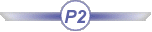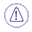This command is only available with the Automotive Body in White Templates product.This task shows how to create a hole, which consists of removing material from a surface.Open the Hole1.CATPart document.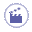1. Click Hole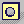.

 The Hole Definition dialog box is displayed.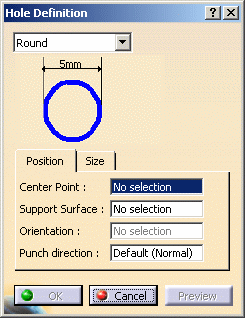Various shapes can be created: round slot (elongated hole) rectangular square The shape is defined on a plane and projected along a direction on the surface. In that case, the nearest projection is used to create the hole.
2. Select the shape in the drop-down list.

3. Click a point to be the Center Point in the geometry or in the specification tree.

4. Select the Support surface.

5. Define the Orientation to align the major axis along a direction.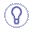You do not need to define an orientation for the round holes.
6. Define the Punch direction.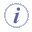If the point lies on the support surface, by default the punch direction is the normal direction at the centre point. If the point does not lie on the support surface, you must define a punch direction.
7. Click Preview.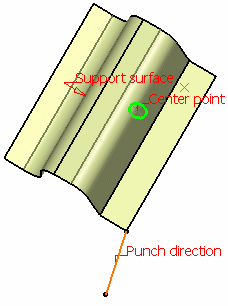8. Define the shape dimensions.

To do so, either :
• click the value to edit (here the rectangular length)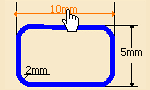The Length dialog box opens to let you modify the dimension.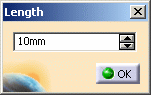• click the Size tab.

 All dimensions related to the selected shape are displayed and can be modified.Some values must be greater than 0mm:
• Diameter for the round hole
• Length and width for the slot and rectangle hole
• Length for the square holeSquare and rectangle holes can be created with a null radius value: in this case, the hole is created without corner.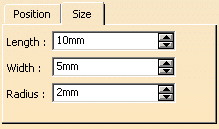Here are the parameters to be defined depending on the shape hole:

 Length Width Radius Round X Slot X X Rectangular X X X Square X X
9. Click OK to create the hole.

 The element (identified as Type Hole.xxx) is added to the specification tree. Round hole Radius = 35mm, Punch Dir. = Default Slot hole Length = 30mm, Width = 15mm Orientation = zx plane, Punch Dir. = Line.1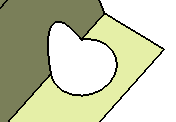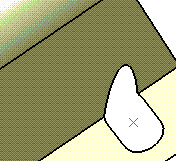Rectangular hole Length = 40mm, Width = 10mm, Radius = 5mm Orientation = yz plane, Punch Dir. = Default Square hole Length = 15mm, Radius = 2mm, Punch Dir. = xy plane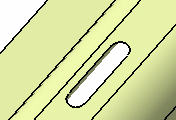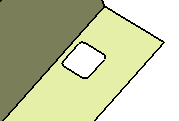If several solutions are possible, the solution with the minimum distance is chosen. If all distances are equal, an error message is issued, asking you to modify the point position.